# Autocorrelation functions of charge dipole moments in DL_MESO_DPD¶

## Purpose of Module¶

This module, gen_dipoleaf.f90, is a generalization of the dipoleaf.f90 post-processing utility of DL_MESO_DPD, the Dissipative Particle Dynamics (DPD) code from the DL_MESO package.

It processes the trajectory (HISTORY) files to obtain the charge dipole moments of all the (neutral) molecules in the system, and subsequently computes the dipole autocorrelation functions (DAFs) for each molecule type. It produces a file DIPAFDAT containing both the un-normalized and normalized DAFs, and, optionally, a file DIPAFFFT containing the Fourier transform (FT) of the latter.

The module can be applied to systems including molecules with a generic charge structure, as long as each molecule is neutral (otherwise the charge dipole moment would be frame-dependent) .

CAVEAT: this module only analyzes molecular trajectories. If a charged molecule is present, an error message will be given, while unbonded charges will not be detected and erroneous results may be obtained. Therefore please keep in mind to not apply this module to systems with unbonded charges.

The charge dipole moment of a neutral molecule is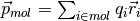where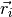are the bead positions and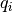their charges. The total charge dipole moment of the simulated volume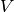is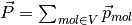. If more than one molecular species are present, one can split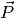into the different species contributions: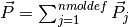.

Given a scalar quantity A, its non-normalized autocorrelation function (AF) is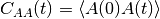, where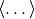indicates an average over trajectories. The normalized one is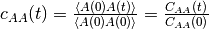.

Here for the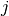-th molecular species we replace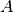with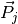, and the product with a scalar product. In this case the average over trajectories translates into a sum over different time origins.

The output file DIPAFDAT contains the DAFs for each molecular species and, at the end of the file, the DAF for the system total charge dipole moment. The output file DIPAFFFT contains the FT of these functions, in the same order.

More in detail, the header of the file DIPAFDAT contains the simulation title and a line with the number of snapshots in HISTORY and of those used for the AFs (naf). Then a block follows for each molecule type, started by the {molecule name}, then three columns of data,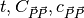. It is intended that in any block only the contribution tofrom a given species is used. The last block is called {all species} and refers to the full system charge dipole moment.

The header of the file DIPAFFFT is as for DIPAFDAT (notice that the number of points for the FT is also set equal to naf). Then a block follows for each molecule type, started by the molecule name, then three columns of data,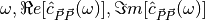, where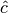is the discrete FT of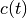.

Possible uses of the output file are: to analyze it to obtain the decay time of autocorrelations, which can be used to define an efficient sampling of the simulation; to compare it with the analogous microscopic one obtained for individual molecules (see Autocorrelation functions of individual charge dipole moments in DL_MESO_DPD).

## Background Information¶

The base code for this module is DL_MESO_DPD, the Dissipative Particle Dynamics code from the mesoscopic simulation package DL_MESO, developed by M. Seaton at Daresbury Laboratory. This open source code is available from STFC under both academic (free) and commercial (paid) licenses. The module is to be used with DL_MESO in its most recently released version, version 2.7 (dating December 2018).

A variant of this module for use with a previous version of DL_MESO, version 2.6 (dating November 2015), can be found in the old-v2.6 directory .

The present module also requires the library FFTW (version 3.x) to be installed.

## Testing¶

The present module gen_dipoleaf.f90 is compiled with the available Fortran 2003 compiler, e.g.:

gfortran -o gen_dipoleaf.exe gen_dipoleaf.f90 -I/usr/local/include -L/usr/local/lib -lfftw3 -lm

where -I indicates the location of the FFTW include file fftw3.f03 and -L points to the directory containing the FFTW library files. The above command gives the most likely locations for these files, although these may need to be adjusted if FFTW has been installed somewhere else on your machine.

The executable must be in the same directory as the HISTORY file to be analyzed. The user is asked to provide the maximum number of snapshots to be used for the AFs (naf) and a switch for the Fourier transform: 1 for yes, 0 (or any other integer) for no.

To input these parameters one can either enter them from the keyboard or write them into a text file (say, input.txt), one per line in the right order, and run the program in this way:

gen_dipoleaf.exe < input.txt

Test: water in oil

As a test, we suggest considering a fluid made of harmonically bonded dimers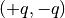. Appropriately fixing the partial charge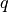and the Bjerrum length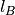, this system mimics water in an oil background as far as the dielectric properties are concerned. For more details about this model, please see the page Test case: a dimer solvent.

Run DL_MESO_DPD using the following CONTROL file:

DL_MESO charged harmonic dimers with dpd repulsion

volume 64.0
temperature 1.0
cutoff 1.0

timestep 0.01
steps 70000
equilibration steps 20000
traj 20000 10
stats every 100
stack size 100
print every 100
job time 7200.0
close time 10.0

ensemble nvt mdvv

ewald sum 1.0 5 5 5
bjerrum 42.0
smear gauss
smear length 0.5 equal

finish


and the FIELD file:

DL_MESO charged harmonic dimers with dpd repulsion

SPECIES 2
solp  1.0   0.46  0
solm  1.0  -0.46  0

MOLECULES 1
DIMER
nummols 96
solp  0.0 0.0 0.0
solm  0.1 0.0 0.0
bonds 1
harm 1 2 5.0 0.0

finish

INTERACTIONS 3
solp  solp  dpd 25.0 1.0 4.5
solm  solm  dpd 25.0 1.0 4.5
solp  solm  dpd 25.0 1.0 4.5

CLOSE


Analyzing the HISTORY file with gen_dipoleaf.exe choosing naf=100, i.e., using this input.txt:

100
1


this output is printed to the standard output:

 nchist:            0          96
Number of time steps in autocorrelation profile?
100
switch for FFT computation? (1=yes, 0 or any other integer=no)
1


The first line shows the histogram of cluster sizes: in this case, it correctly gives 96 molecules of two beads. Since internally the module checks that each molecule is a connected cluster , this line should always give a histogram with the molecule sizes (shown up to the maximum number of beads per molecule).

The DIPAFDAT file is (only the first fifteen lines are shown):

DL_MESO charged harmonic dimers with dpd repulsion
5001       100

DIMER
0.000000E+00  1.462829E+01  1.000000E+00
1.000000E-01  1.403865E+01  9.596923E-01
2.000000E-01  1.258271E+01  8.601627E-01
3.000000E-01  1.074853E+01  7.347772E-01
4.000000E-01  8.870221E+00  6.063746E-01
5.000000E-01  7.113151E+00  4.862601E-01
6.000000E-01  5.559272E+00  3.800358E-01
7.000000E-01  4.250179E+00  2.905452E-01
8.000000E-01  3.187677E+00  2.179119E-01
9.000000E-01  2.349055E+00  1.605831E-01


and the DIPAFFFT file is (only the first fifteen lines are shown):

DL_MESO charged harmonic dimers with dpd repulsion
5001       100

DIMER
0.000000E+00  5.757016E+00  0.000000E+00
6.283185E-01  5.682940E+00 -1.682430E+00
1.256637E+00  4.084017E+00 -2.585684E+00
1.884956E+00  3.921155E+00 -3.116275E+00
2.513274E+00  3.381181E+00 -3.800208E+00
3.141593E+00  2.320091E+00 -3.074025E+00
3.769911E+00  1.751324E+00 -3.126105E+00
4.398230E+00  1.443640E+00 -2.653086E+00
5.026548E+00  1.105522E+00 -2.423820E+00
5.654867E+00  9.472693E-01 -2.056408E+00


Below we show a plot of the normalized AF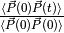(obtained using the first and third columns of DIPAFDAT)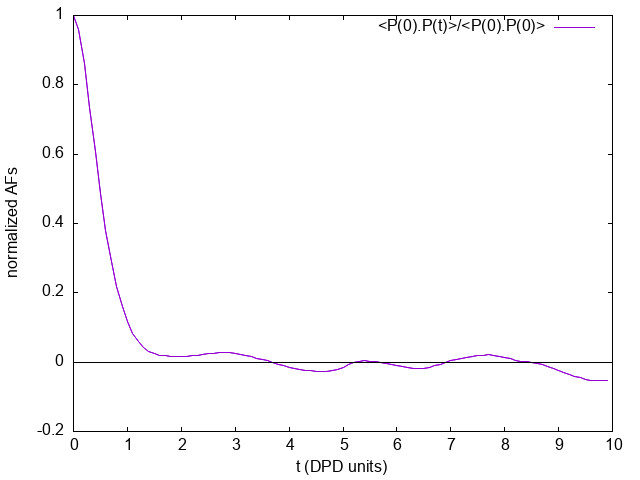## Source Code¶

  1 2 3 4 5 6 7 8 9 10 11 12 13 14 15 16 17 18 19 20 21 22 23 24 25 26 27 28 29 30 31 32 33 34 35 36 37 38 39 40 41 42 43 44 45 46 47 48 49 50 51 52 53 54 55 56 57 58 59 60 61 62 63 64 65 66 67 68 69 70 71 72 73 74 75 76 77 78 79 80 81 82 83 84 85 86 87 88 89 90 91 92 93 94 95 96 97 98 99 100 101 102 103 104 105 106 107 108 109 110 111 112 113 114 115 116 117 118 119 120 121 122 123 124 125 126 127 128 129 130 131 132 133 134 135 136 137 138 139 140 141 142 143 144 145 146 147 148 149 150 151 152 153 154 155 156 157 158 159 160 161 162 163 164 165 166 167 168 169 170 171 172 173 174 175 176 177 178 179 180 181 182 183 184 185 186 187 188 189 190 191 192 193 194 195 196 197 198 199 200 201 202 203 204 205 206 207 208 209 210 211 212 213 214 215 216 217 218 219 220 221 222 223 224 225 226 227 228 229 230 231 232 233 234 235 236 237 238 239 240 241 242 243 244 245 246 247 248 249 250 251 252 253 254 255 256 257 258 259 260 261 262 263 264 265 266 267 268 269 270 271 272 273 274 275 276 277 278 279 280 281 282 283 284 285 286 287 288 289 290 291 292 293 294 295 296 297 298 299 300 301 302 303 304 305 306 307 308 309 310 311 312 313 314 315 316 317 318 319 320 321 322 323 324 325 326 327 328 329 330 331 332 333 334 335 336 337 338 339 340 341 342 343 344 345 346 347 348 349 350 351 352 353 354 355 356 357 358 359 360 361 362 363 364 365 366 367 368 369 370 371 372 373 374 375 376 377 378 379 380 381 382 383 384 385 386 387 388 389 390 391 392 393 394 395 396 397 398 399 400 401 402 403 404 405 406 407 408 409 410 411 412 413 414 415 416 417 418 419 420 421 422 423 424 425 426 427 428 429 430 431 432 433 434 435 436 437 438 439 440 441 442 443 444 445 446 447 448 449 450 451 452 453 454 455 456 457 458 459 460 461 462 463 464 465 466 467 468 469 470 471 472 473 474 475 476 477 478 479 480 481 482 483 484 485 486 487 488 489 490 491 492 493 494 495 496 497 498 499 500 501 502 503 504 505 506 507 508 509 510 511 512 513 514 515 516 517 518 519 520 521 522 523 524 525 526 527 528 529 530 531 532 533 534 535 536 537 538 539 540 541 542 543 544 545 546 547 548 549 550 551 552 553 554 555 556 557 558 559 560 561 562 563 564 565 566 567 568 569 570 571 572 573 574 575 576 577 578 579 580 581 582 583 584 585 586 587 588 589 590 591 592 593 594 595 596 597 598 599 600 601 602 603 604 605 606 607 608 609 610 611 612 613 614 615 616 617 618 619 620 621 622 623 624 625 626 627 628 629 630 631 632 633 634 635 636 637 638 639 640 641 642 643 644 645 646 647 648 649 650 651 652 653 654 655 656 657 658 659 660 661 662 663 664 665 666 667 668 669 670 671 672 673 674 675 676 677 678 679 680 681 682 683 684 685 686 687 688 689 690 691 692 693 694 695 696 697 698 699 700 701 702 703 704 705 706 707 708 709 710 711 712 713 714 715 716 717 718 719 720 721 722 723 724 725 726 727 728 729 730 731 732 733 734 735 PROGRAM gen_dipoleaf !************************************************************************************* ! module to compute autocorrelation functions of charge dipole moments in DL_MESO_DPD ! ! authors: m. a. seaton and s. chiacchiera, March 2017 (amended August 2017, January ! 2021) !************************************************************************************* USE, INTRINSIC :: iso_c_binding IMPLICIT none INTEGER, PARAMETER :: dp = SELECTED_REAL_KIND (15, 307) INTEGER, PARAMETER :: li = SELECTED_INT_KIND (12) INTEGER, PARAMETER :: ntraj=10 INTEGER, PARAMETER :: endversion = 1 REAL(KIND=dp), PARAMETER :: pi=3.141592653589793_dp CHARACTER(80) :: text CHARACTER(8), ALLOCATABLE :: namspe (:), nammol (:) INTEGER, ALLOCATABLE :: ltp (:), ltm (:), mole (:), bndtbl (:,:) INTEGER, ALLOCATABLE :: nbdmol (:), readint (:) INTEGER, ALLOCATABLE :: visit (:), from (:) INTEGER :: nrtout INTEGER :: chain, imol, ioerror, i, numtraj, j, k, l, nmoldef, ibond, nbdmolmx INTEGER :: nspe, nbeads, nusyst, nmbeads, nsyst, numbond, global, species, molecule INTEGER :: nummol, lfrzn, rnmol, keytrj, srfx, srfy, srfz INTEGER :: naf, nsamp, n1 INTEGER :: endver, Dlen, nstep, framesize, lend, leni INTEGER(KIND=li) :: filesize, mypos, currentpos, lend_li, leni_li, framesizeli, numbeadsli REAL(KIND=dp), ALLOCATABLE :: xxx (:), yyy (:), zzz (:), readdata (:) REAL(KIND=dp), ALLOCATABLE :: nmol (:), chg (:), molchg (:) REAL(KIND=dp), ALLOCATABLE :: dipx_box (:), dipy_box (:), dipz_box (:) REAL(KIND=dp), ALLOCATABLE :: dipdata (:,:,:), dipdata_box (:,:), corrdata (:) REAL(KIND=dp) :: dimx, dimy, dimz, shrdx, shrdy, shrdz REAL(KIND=dp) :: amass, rcii REAL(KIND=dp) :: time REAL(KIND=dp) :: domega, dt, time0 REAL(KIND=dp) :: dx0, dy0, dz0 INTEGER :: nftpts COMPLEX(C_DOUBLE_COMPLEX), ALLOCATABLE :: fftdata (:) LOGICAL :: eof, lfft, swapend, bigend ! determine number of bytes for selected double precision and integer kinds ! (the default SELECTED_REAL_KIND (15, 307) should return 8 bytes) lend = STORAGE_SIZE (1.0_dp) / 8 leni = BIT_SIZE (1) / 8 lend_li = INT (lend, KIND=li) leni_li = INT (leni, KIND=li) ! check endianness of machine bigend = (IACHAR(TRANSFER(1,"a"))==0) ! determine if HISTORY file exists, which endianness to use, ! if type of real is correct INQUIRE (file = 'HISTORY', EXIST = eof) IF (.NOT. eof) THEN PRINT *, "ERROR: cannot find HISTORY file" STOP END IF OPEN (ntraj, file = 'HISTORY', access = 'stream', form = 'unformatted', status = 'unknown') swapend = .false. READ (ntraj) endver, Dlen IF (endver/=endversion) THEN swapend = .true. CLOSE (ntraj) IF (bigend) THEN OPEN (ntraj, file = 'HISTORY', access = 'stream', form = 'unformatted', status = 'unknown', convert = 'little_endian') ELSE OPEN (ntraj, file = 'HISTORY', access = 'stream', form = 'unformatted', status = 'unknown', convert = 'big_endian') END IF READ (ntraj) endver, Dlen IF (endver/=endversion) THEN PRINT *, "ERROR: corrupted HISTORY file or created with incorrect version of DL_MESO" STOP END IF END IF IF (Dlen/=lend) THEN PRINT *, "ERROR: incorrect type of real number used in HISTORY file" PRINT *, " recompile gen_dipoleaf.f90 with reals of ", Dlen, " bytes" STOP END IF ! read file size, number of frames and timestep numbers READ (ntraj) filesize, numtraj, nstep ! Read where the number of beads, molecules and bonds are determined ! Arrays are filled with names of particles and molecules READ (ntraj) text READ (ntraj) nspe, nmoldef, nusyst, nsyst, numbond, keytrj, srfx, srfy, srfz IF (numbond==0) THEN PRINT *, 'ERROR: no molecules in trajectory data!' STOP END IF IF (srfx>1 .OR. srfy>1 .OR. srfz>1) THEN WRITE (*,*) "ERROR: Hard walls, electrostatics not implemented in DL_MESO_DPD yet!" STOP END IF IF (srfx==1 .OR. srfy==1 .OR. srfz==1) THEN WRITE (*,*) "ERROR: Systems under shear not yet implemented!" STOP END IF framesize = (keytrj+1) * 3 ALLOCATE (readint (1:nsyst), readdata (1:framesize)) ! get number of beads to be tracked when reading trajectory file (molecular beads) nmbeads = nsyst - nusyst ALLOCATE (namspe (nspe), nammol (nmoldef)) ALLOCATE (xxx (1:nmbeads), yyy (1:nmbeads), zzz (1:nmbeads)) ALLOCATE (ltp (1:nmbeads), ltm (1:nmbeads), mole (1:nmbeads)) ALLOCATE (nmol (1:nmoldef), nbdmol (1:nmoldef)) ALLOCATE (chg (nspe)) ALLOCATE (bndtbl (numbond, 2)) ALLOCATE (visit (nmbeads), from (nmbeads)) DO i = 1, nspe READ (ntraj) namspe (i), amass, rcii, chg (i), lfrzn END DO DO i = 1, nmoldef READ (ntraj) nammol (i) END DO ! reading of bead species and molecule types nummol = 0 !counter for number of molecules ibond = 0 !counter for bonds DO i = 1, nsyst READ (ntraj) global, species, molecule, chain IF (global>nusyst .AND. global<=nsyst) THEN ltp (global-nusyst) = species ltm (global-nusyst) = molecule mole (global-nusyst) = chain nummol = MAX (nummol, chain) END IF END DO ! reading of bond tables IF (numbond>0) THEN DO i = 1, numbond READ (ntraj) bndtbl (i, 1), bndtbl (i, 2) END DO END IF bndtbl = bndtbl - nusyst ! reached end of header: find current position in file INQUIRE (unit=ntraj, POS=currentpos) ! find timestep size from times in first two frames framesizeli = INT (framesize, KIND=li) numbeadsli = INT (nsyst, KIND=li) READ (ntraj, IOSTAT=ioerror, POS=currentpos) time0 mypos = currentpos + (numbeadsli + 1_li) * leni_li + (framesizeli * numbeadsli + 7_li) * lend_li READ (ntraj, IOSTAT=ioerror, POS=mypos) time dt = time - time0 ! determine numbers of molecules and beads per molecule type nmol = 0.0_dp nbdmol = 0 chain = 0 imol = 0 !necessary to avoid out of bounds DO i = 1, nmbeads IF (mole (i) /= chain) THEN chain = mole (i) imol = ltm (i) nmol (imol) = nmol (imol) + 1.0_dp END IF IF (imol > 0) nbdmol (imol) = nbdmol (imol) + 1 END DO DO i = 1, nmoldef rnmol = NINT (nmol (i)) IF (rnmol>0) THEN nbdmol (i) = nbdmol (i) / rnmol END IF END DO nbdmolmx = MAXVAL (nbdmol (1:nmoldef)) ! obtain connectivity information (needed only once) CALL connect (nmbeads, numbond, bndtbl, nbdmolmx, visit, from) !Checking for charge neutrality of all molecules ALLOCATE (molchg (nummol)) molchg (:) = 0._dp DO i = 1, nmbeads imol = mole (i) molchg (imol) = molchg (imol) + chg (ltp (i)) END DO DO i = 1, nummol IF (ABS (molchg (i)) > 1.0e-16_dp) THEN WRITE (*,*) "molecule number",i," is not neutral! (The dipole moment is frame-dependent)" WRITE (*,*) "its charge is=", molchg (i) WRITE (*,*) "its type is=", nammol (i) STOP ENDIF END DO CALL check_molecules !checks that beads are labelled as expected ! Get the maximum number of time steps for autocorrelation WRITE (*,*) "Number of time steps in autocorrelation profile? " READ (*,*) naf IF (naf<1 .OR. naf>numtraj) naf = numtraj ! Get the switch for FFT computation WRITE (*,*) "switch for FFT computation? (1=yes, 0 or any other integer=no)" READ (*,*) n1 lfft = (n1 == 1) !reading trajectories and computing charge dipole moments ALLOCATE (dipdata (4, nmoldef, numtraj)) ALLOCATE (dipx_box (nmoldef), dipy_box (nmoldef), dipz_box (nmoldef)) eof = .false. DO k = 1, numtraj mypos = currentpos + INT (k-1, KIND=li) * ((numbeadsli + 1_li) * leni_li + (framesizeli * numbeadsli + 7_li) * lend_li) READ (ntraj, POS = mypos, IOSTAT=ioerror) time, nbeads, dimx, dimy, dimz, shrdx, shrdy, shrdz IF (ioerror/=0) THEN eof = .true. IF (k==1) THEN PRINT *, 'ERROR: cannot find trajectory data in HISTORY files' STOP END IF EXIT END IF READ (ntraj) readint (1:nsyst) DO i = 1, nsyst global = readint (i) READ (ntraj) readdata (1:framesize) IF (global>nusyst .AND. global<=nsyst) THEN xxx (global-nusyst) = readdata (1) yyy (global-nusyst) = readdata (2) zzz (global-nusyst) = readdata (3) END IF END DO CALL compute_charge_dipoles (dipx_box, dipy_box, dipz_box) ! the dipole components are stored for all the snapshots DO j = 1, nmoldef dipdata (1, j, k) = dipx_box (j) dipdata (2, j, k) = dipy_box (j) dipdata (3, j, k) = dipz_box (j) dipdata (4, j, k) = time END DO END DO ! end of loop over trajectories IF (k <= numtraj) THEN WRITE (*,*) "ERROR: problem with the number of snapshots!" STOP END IF ! Close the trajectory file CLOSE (ntraj) nsamp = numtraj - naf + 1 ALLOCATE (corrdata (naf)) ! define FFT size if needed IF (lfft) THEN nftpts = naf ! modify here to change the size of the DFT domega = 2.0_dp * pi / (dt * REAL(nftpts, KIND=dp)) ALLOCATE (fftdata (nftpts)) END IF ! Open output file, compute the autocorrelation and write it there nrtout = ntraj + 1 IF (numtraj>0) THEN OPEN (nrtout, file='DIPAFDAT', status='replace') WRITE (nrtout, '(a80)') text WRITE (nrtout, '(2i10)') numtraj,naf WRITE (nrtout, '(/)') ! Open the FT otuput file if needed IF (lfft) THEN OPEN (nrtout+1, file='DIPAFFFT', status='replace') WRITE (nrtout+1, '(a80)') text WRITE (nrtout+1, '(2i10)') numtraj,nftpts WRITE (nrtout+1, '(/)') END IF DO j = 1, nmoldef corrdata = 0.0_dp WRITE (nrtout,'(a8)') nammol (j) IF (lfft) WRITE (nrtout+1,'(a8)') nammol (j) DO i = 1, nsamp dx0 = dipdata (1, j, i) dy0 = dipdata (2, j, i) dz0 = dipdata (3, j, i) DO l = 1, naf corrdata (l) = corrdata (l) + dipdata (1, j, i+l-1) * dx0 + dipdata (2, j, i+l-1) * dy0 & + dipdata (3, j, i+l-1) * dz0 END DO END DO corrdata = corrdata / REAL (nsamp, KIND=dp) DO i = 1, naf WRITE (nrtout, '(1p,3e14.6)') REAL (i-1, KIND=dp)*dt, corrdata (i), corrdata (i)/corrdata(1) END DO WRITE (nrtout, '(/)') IF (lfft) THEN fftdata (:) = corrdata (:)/ corrdata (1) ! adapt here if nftpts differs from naf CALL fft (fftdata) DO i = 1, nftpts WRITE (nrtout+1, '(1p,3e14.6)') REAL (i-1, KIND=dp)*domega, fftdata (i) END DO WRITE (nrtout+1, '(/)') END IF END DO ! Calculation for the total system dipole ALLOCATE (dipdata_box (4, numtraj)) dipdata_box = SUM (dipdata, 2) !sum of dipoles over all molecular species corrdata = 0.0_dp WRITE (nrtout, '("all species")') IF (lfft) WRITE (nrtout+1, '("all species")') DO i = 1, nsamp dx0 = dipdata_box (1, i) dy0 = dipdata_box (2, i) dz0 = dipdata_box (3, i) DO l = 1, naf corrdata (l) = corrdata (l) + dipdata_box (1, i+l-1) * dx0 + dipdata_box (2, i+l-1) * dy0 & + dipdata_box (3, i+l-1) * dz0 END DO END DO corrdata = corrdata / REAL (nsamp, KIND=dp) DO i = 1, naf WRITE (nrtout, '(1p,3e14.6)') REAL (i-1, KIND=dp)*dt, corrdata (i), corrdata (i)/corrdata(1) END DO WRITE (nrtout, '(/)') IF (lfft) THEN fftdata (:) = corrdata (:)/ corrdata (1) ! adapt here if nftpts differs from naf call fft (fftdata) DO i = 1, nftpts WRITE (nrtout+1, '(1p,3e14.6)') REAL (i-1, KIND=dp)*domega, fftdata (i) END DO WRITE (nrtout+1, '(/)') END IF DEALLOCATE (dipdata_box) END IF ! Close the output files CLOSE (nrtout) IF (lfft) CLOSE (nrtout+1) DEALLOCATE (readint, readdata) DEALLOCATE (namspe, nammol) DEALLOCATE (xxx, yyy, zzz) DEALLOCATE (ltp, ltm, mole) DEALLOCATE (nmol, nbdmol) DEALLOCATE (chg, molchg) DEALLOCATE (dipx_box, dipy_box, dipz_box) DEALLOCATE (bndtbl) DEALLOCATE (visit, from) DEALLOCATE (dipdata, corrdata) IF (lfft) DEALLOCATE (fftdata) CONTAINS SUBROUTINE check_molecules !************************************************************************************* ! subroutine to check molecular content and labelling ! ! authors: s. chiacchiera, February 2017 !************************************************************************************* IMPLICIT NONE INTEGER i, j, k, tm, tp, imol, im, ibd INTEGER mxmolsize INTEGER, ALLOCATABLE :: molbeads (:,:) mxmolsize = 0 DO i = 1, nmoldef mxmolsize = MAX (nbdmol(i), mxmolsize) END DO ALLOCATE (molbeads (nmoldef, mxmolsize)) molbeads (:,:) = 0 imol = 0 ibd = 0 DO i = 1, nmoldef DO j = 1, NINT (nmol(i)) imol = imol +1 DO k = 1, nbdmol(i) ibd = ibd +1 tm = ltm (ibd) tp = ltp (ibd) im = mole (ibd) IF (j==1) THEN molbeads (i, k) = tp ELSE IF (molbeads (i, k) /= tp) THEN WRITE (*,*) "ERROR: Problem with molecular content!" STOP ENDIF ENDIF IF (tm/=i.OR.im/=imol)THEN WRITE (*,*) "ERROR: Problem with molecules labels!" STOP ENDIF END DO END DO END DO IF (imol/=nummol) THEN WRITE (*,*) "ERROR: imol and nummol differ!" STOP ENDIF DEALLOCATE (molbeads) RETURN END SUBROUTINE check_molecules SUBROUTINE compute_charge_dipoles (dipx_box, dipy_box, dipz_box) !************************************************************************************* ! subroutine to compute charge dipole moments ! ! authors: m. a. seaton and s. chiacchiera, February 2017 ! ! input: xxx, yyy, zzz (at a given time step) and chg ! input: visit and from (obtained using connect) ! output: the x,y,z components of the total dipole, for each molecule type (at a given ! time step) ! ! (NB: this is a slightly modified version, with fewer outputs) !************************************************************************************* IMPLICIT NONE INTEGER i, j, k, tm, tp, imol, ibd, count, ipr REAL(KIND=dp), DIMENSION(nmoldef) :: dipx_box, dipy_box, dipz_box REAL(KIND=dp) :: x, y, z, dx, dy, dz, xpre, ypre, zpre REAL(KIND=dp) :: dipx, dipy, dipz REAL(KIND=dp), DIMENSION(nmbeads) :: xabs, yabs, zabs dipx_box (:) = 0._dp dipy_box (:) = 0._dp dipz_box (:) = 0._dp imol = 0 count = 0 ! xabs = 0._dp ! just to keep it clean ! yabs = 0._dp ! zabs = 0._dp DO i = 1, nmoldef tm = i DO j = 1, NINT (nmol(i)) imol = imol + 1 dipx = 0._dp ! dipole of a SINGLE molecule dipy = 0._dp dipz = 0._dp DO k = 1, nbdmol(i) count = count + 1 ibd = visit (count) ipr = from (count) IF (ipr /= 0) THEN xpre = xabs (ipr) ypre = yabs (ipr) zpre = zabs (ipr) ELSE IF (k == 1) THEN xpre = 0._dp ypre = 0._dp zpre = 0._dp ELSE WRITE (*,*) "Unconnected molecule!" STOP ENDIF ENDIF tp = ltp (ibd) dx = xxx (ibd) - xpre dy = yyy (ibd) - ypre dz = zzz (ibd) - zpre dx = dx - dimx * ANINT (dx/dimx) dy = dy - dimy * ANINT (dy/dimy) dz = dz - dimz * ANINT (dz/dimz) x = xpre + dx y = ypre + dy z = zpre + dz dipx = dipx + x * chg (tp) dipy = dipy + y * chg (tp) dipz = dipz + z * chg (tp) xabs (ibd) = x yabs (ibd) = y zabs (ibd) = z END DO dipx_box (tm) = dipx_box (tm) + dipx dipy_box (tm) = dipy_box (tm) + dipy dipz_box (tm) = dipz_box (tm) + dipz END DO END DO IF (imol/=nummol) THEN WRITE (*,*) "ERROR: imol and nummol differ!" STOP ENDIF RETURN END SUBROUTINE compute_charge_dipoles SUBROUTINE fft (x) !************************************************************************************* ! Subroutine to call FFTW (v3) one-dimensional complex DFT. ! Notice that the input array is overwritten with the its Discrete Fourier Transform. ! ! author: s. chiacchiera, August 2017 ! amended: m. a. seaton, January 2021 !************************************************************************************* USE, INTRINSIC :: iso_c_binding IMPLICIT none INCLUDE 'fftw3.f03' COMPLEX(C_DOUBLE_COMPLEX), INTENT(INOUT) :: x (:) INTEGER :: n TYPE(C_PTR) :: plan n = SIZE (x) plan = fftw_plan_dft_1d (n, x, x, FFTW_FORWARD, FFTW_ESTIMATE) CALL fftw_execute_dft (plan, x, x) CALL fftw_destroy_plan (plan) RETURN END SUBROUTINE fft End PROGRAM gen_dipoleaf SUBROUTINE connect (nbeads, nbonds, bndtbl, mxmolsize, visit, from) !********************************************************************** ! Analyzes all the bonds (bndtbl) to obtain a schedule (visit, from) ! to visit the beads so that each cluster is visited along a connected ! path. "visit" gives the order to include beads, "from" gives the bead ! to attach them to. ! (Note: vocabulary from infection propagation used to move along ! clusters) ! ! author: s. chiacchiera, February 2017 ! amended: m. a. seaton, January 2021 !********************************************************************** IMPLICIT none INTEGER, INTENT (IN) :: bndtbl (nbonds,2) INTEGER, INTENT (IN) :: nbeads, nbonds INTEGER, INTENT (IN) :: mxmolsize INTEGER :: ic, i, k, nn, nclu, nper, lab, ref, count INTEGER, ALLOCATABLE :: firstnn (:), lastnn (:), deg (:) INTEGER, ALLOCATABLE :: labnn (:) INTEGER, ALLOCATABLE :: state (:) INTEGER, ALLOCATABLE :: perlab (:), perref (:) INTEGER, ALLOCATABLE :: nchist (:) INTEGER, INTENT (OUT) :: visit (nbeads), from (nbeads) ALLOCATE (firstnn (nbeads), lastnn (nbeads), deg (nbeads), labnn (2*nbonds)) ALLOCATE (state (nbeads)) ALLOCATE (perlab (nbeads), perref (nbeads)) ALLOCATE (nchist (mxmolsize)) !----------------------------------------------------------------------- CALL organize (nbeads, nbonds, labnn, firstnn, lastnn, deg) !----------------------------------------------------------------------- state (:) = 0 nchist (:) = 0 visit (:) = 0 from (:) = 0 count = 0 !----------------------------------------------------------------------- ic = 0 !----------------------------------------------------------------------- DO WHILE (ic < nbeads) ! ic = label of bead used to "grow" a cluster ic = ic + 1 IF( state (ic) /= 0) THEN WRITE (*,*) "ERROR: labels are not as expected!" STOP END IF nclu = 1 count = count + 1 visit (ic) = ic IF (deg (ic) == 0) THEN state (ic) = -1 IF (nclu <= mxmolsize) nchist (nclu) = nchist (nclu) +1 CYCLE END IF state (ic) = 1 ! ic is "infected" ! nearest neighbours of ic are marked as "goint to be infected" -> a.k.a. perimeter nper = 0 perlab (:) = 0 perref (:) = 0 DO k = firstnn (ic), lastnn (ic) nn = labnn (k) IF( state (nn) /= 0) THEN WRITE (*,*) "ERROR: labels are not as expected!" STOP END IF nper = nper + 1 perlab (nper) = nn !new bead in perimeter perref (nper) = ic !its reference bead (origin of the link) state (nn) = 2 END DO state (ic) = 3 ! ic is "dead" DO WHILE (nper > 0) i = 1 ! pick a bead of "perimeter" to be analyzed lab = perlab (i) ref = perref (i) perlab (i) = perlab (nper) perref (i) = perref (nper) nper = nper - 1 IF (state (lab) == 3) THEN CYCLE END IF state (lab) = 1 ! "lab" is added to the cluster nclu = nclu + 1 count = count + 1 visit (count) = lab from (count) = ref DO k = firstnn (lab), lastnn (lab) ! check nn of newly added nn = labnn (k) IF( (state (nn) == 2) .OR. (state (nn) == 3)) CYCLE nper = nper + 1 perlab (nper) = nn !new bead in perimeter perref (nper) = lab !its reference bead (origin of the link) state (nn) = 2 END DO state (lab) = 3 END DO nchist (nclu) = nchist (nclu) +1 ic = ic + nclu - 1 ! prepare ic for the next cluster END DO WRITE (*,*) "nchist: ", nchist !----------------------------------------------------------------------- DEALLOCATE (firstnn, lastnn, deg, labnn) DEALLOCATE (state) DEALLOCATE (perlab, perref) DEALLOCATE (nchist) RETURN !----------------------------------------------------------------------- CONTAINS !----------------------------------------------------------------------- SUBROUTINE organize (N, NL, labnn, firstnn, lastnn, deg) !********************************************************************** ! Analyzes the bonds (bndtbl) to obtain the degree (=number of bonds) ! of each bead, and the nearest neighbours list. ! N in the number of beads (vertices) and NL of bonds (links). ! ! author: s. chiacchiera, February 2017 !********************************************************************** IMPLICIT none INTEGER, INTENT(IN) :: N, NL INTEGER :: i,l,count_lab, i1,i2 INTEGER, DIMENSION (N), INTENT(OUT) :: deg INTEGER, DIMENSION (N), INTENT(OUT) :: firstnn, lastnn INTEGER, DIMENSION (2*NL), intent(OUT) :: labnn deg(:)=0 firstnn(:)=0 lastnn(:)=0 labnn(:)=0 count_lab=0 DO i=1,N DO l=1,NL IF(bndtbl(l,1).EQ.i)THEN deg(i)=deg(i)+1 count_lab=count_lab+1 labnn(count_lab)=bndtbl(l,2) ENDIF IF(bndtbl(l,2).EQ.i)THEN deg(i)=deg(i)+1 count_lab=count_lab+1 labnn(count_lab)=bndtbl(l,1) ENDIF END DO END DO i1=1 i2=0 DO i=1,N IF (deg (i)==0) CYCLE firstnn(i)=i1 i2=i1+deg(i)-1 lastnn(i)=i2 i1=i2+1 END DO RETURN END SUBROUTINE organize !----------------------------------------------------------------------- END SUBROUTINE connect 
  (1, 2) Disambiguation on the concept of molecule. In DL_MESO a defined molecule is a set of beads that can be bonded together or not. For the purpose of this module it is required that each molecule is a connected cluster (via stretching bonds). In fact, this - together with the reasonable assumption that each stretching bond cannot be stretched to more than half the system linear size - allows us to univocally define the charge dipole moment of each molecule.
  M. P. Allen and D. J. Tildesley, “Computer simulation of liquids”, Oxford University Press, Oxford (1987).
  A small change to specifying charge smearing schemes and lengths in CONTROL files has been made since version 2.6: the old-v2.6 folder includes the CONTROL file for the test shown here that will work with this version of DL_MESO.